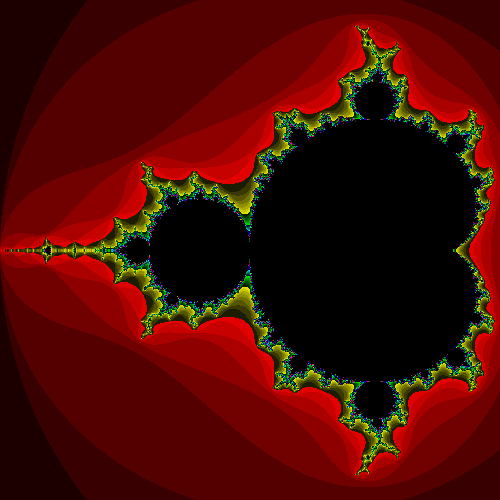## The Mandelbrot Set

For those of you already familiar with the Mandelbrot Set, I suppose this will be like visiting with an old friend. For those of you who aren’t — you’re in for a treat!Okay, you say, that looks pretty cool I guess, but…huh? Well, to answer the fundamental question of “huh?” we need to dust off our Complex Number Skills. (If you don’t know what a complex number is — or need a refresher — read my explanation of complex numbers.)

Here’s what you do. Pick some complex number c and define the function$f(z) = z^2 + c$

(Note that z often denotes a complex number.) Now start with$z = 0$ and iterate the function f, by taking each value output from the function and putting it back into the function. In other words, find$f(0), f(f(0)), f(f(f(0))), f(f(f(f(0))))$, and so on. For example, let’s pick$c = 1 + i$ and follow this process for a few steps:$\begin{array}{rcl} f(0) & = & 0^2 + (1 + i) = 1 + i \\ f(f(0)) & = & f(1 + i) = (1 + i)^2 + (1 + i) = 1 + 3i \\ f(f(f(0))) & = & f(1 + 3i) = (1 + 3i)^2 + (1 + i) = \dots \\ & \vdots & \end{array}$

and so on. This is a very simple process, and can be worked out by hand fairly quickly. It can be worked out by a computer in the blink of an eye.

For some values of c, iterating the function f will tend to produce complex numbers that just get bigger and bigger. For other values of c, iterating the function will produce complex numbers that stay relatively small, no matter how long you keep iterating the function. (Of course there are technical definitions corresponding to the phrases “bigger and bigger” and “stay relatively small”, but for now we won’t worry about what they are.) Try starting with the value$c = 0 + 0.1i$ to see an example of the latter.

Well, now we’re ready to define the Mandelbrot set: the Mandelbrot set is the set of all complex numbers c for which iterating the function$f(z) = z^2 + c$ produces complex numbers that stay relatively small.

We can make a picture of the Mandelbrot set by letting each complex number$a + bi$ correspond to the point with coordinates$(a,b)$. For example, a computer can easily make a picture of the Mandelbrot set by looking at each point on the screen one by one, deciding which complex number c that point corresponds to, then (say) coloring the point black if c is in the Mandelbrot set, and white otherwise. (Often, instead of just white, programs will choose different colors for points which are not in the Mandelbrot set, based on how many iterations the program had to do before it could decide whether the point was in the set or not.)

You might think that with such a simple function, the picture would be simple as well — like a circle, or a parabola, or something like that. But in fact you get that crazy thing shown above. Iteration can make even the simplest functions behave in very complex ways!

In fact, the Mandelbrot set is what is known as a fractal, an object which is infinitely detailed and contains copies (or near-copies) of itself on all different scales. This means that (theoretically) you can keep zooming into the Mandelbrot forever, and you will always see details just as fine and complex as you do at the “top level”. Moreover, as you zoom in, you will find structures that appear to be tiny copies of the entire picture.

But don’t take my word for it — here are some nice zoomed-in pictures of the Mandelbrot set, and you can find lots more with Google image search. You might also want to download some software for viewing fractals to be able to play around with it yourself.

The most amazing thing is that no one made up these pictures — they have existed forever, built into the mathematical structure of the universe, just waiting for someone to come along and iterate a certain function and make a picture out of it. And in fact, it’s only been since the invention of computers that we’ve been able to do such things (although it’s easy to carry out the iteration described above for a particular value of c by hand, to do it for enough different values of c to make a decent picture would take so long, and be so mind-numbingly tedious, as to make it practically impossible.)

More about the Mandelbrot set, if you’re interested: